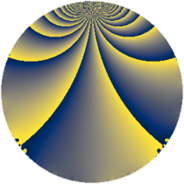# Properties

 Label 1386.2.nLevel $1386$ Weight $2$ Character orbit 1386.n Rep. character $\chi_{1386}(439,\cdot)$ Character field $\Q(\zeta_{6})$ Dimension $192$ Sturm bound $576$

# Related objects

## Defining parameters

 Level: $$N$$ $$=$$ $$1386 = 2 \cdot 3^{2} \cdot 7 \cdot 11$$ Weight: $$k$$ $$=$$ $$2$$ Character orbit: $$[\chi]$$ $$=$$ 1386.n (of order $$6$$ and degree $$2$$) Character conductor: $$\operatorname{cond}(\chi)$$ $$=$$ $$693$$ Character field: $$\Q(\zeta_{6})$$ Sturm bound: $$576$$

## Dimensions

The following table gives the dimensions of various subspaces of $$M_{2}(1386, [\chi])$$.

Total New Old
Modular forms 592 192 400
Cusp forms 560 192 368
Eisenstein series 32 0 32

## Trace form

 $$192q + 96q^{4} + 8q^{9} + O(q^{10})$$ $$192q + 96q^{4} + 8q^{9} + 8q^{11} + 8q^{15} - 96q^{16} + 24q^{23} - 192q^{25} + 24q^{26} - 36q^{27} + 18q^{33} - 8q^{36} + 24q^{42} + 4q^{44} - 32q^{53} + 24q^{58} + 60q^{59} + 16q^{60} - 192q^{64} - 12q^{70} + 48q^{71} - 60q^{75} + 14q^{77} + 32q^{78} - 96q^{81} + 16q^{86} - 60q^{89} + 12q^{92} + 72q^{93} + 46q^{99} + O(q^{100})$$

## Decomposition of $$S_{2}^{\mathrm{new}}(1386, [\chi])$$ into newform subspaces

The newforms in this space have not yet been added to the LMFDB.

## Decomposition of $$S_{2}^{\mathrm{old}}(1386, [\chi])$$ into lower level spaces

$$S_{2}^{\mathrm{old}}(1386, [\chi]) \cong$$ $$S_{2}^{\mathrm{new}}(693, [\chi])$$$$^{\oplus 2}$$